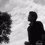# Hyperbolic integral

Here on facebook timeline Sir Srinivasa Raghava share a integral problem

1. $\text{Prove}$ $\int_0^{\infty}\left(\tanh\left(\frac{x}{1}\right)+\tanh\left(\frac{x}{2}\right)+\tanh\left(\frac{x}{3}\right)+\tanh\left(\frac{x}{4}\right)\right)\frac{e^{-x}}{x}dx$$=\log\left(\frac{33554432\Gamma\left(\frac{13}{12}\right)^2\Gamma\left(\frac{17}{12}\right)\Gamma\left(\frac{7}{4}\right)^8}{225\pi^4\Gamma\left(\frac{7}{12}\right)^2\Gamma\left(\frac{11}{12}\right)^2}\right)^{\frac{1}{3}}=\log(6)=\log(2)+\log(3)$

So this inspires me to generalize integral in closed form $\int_{0}^{\infty} \left( \tanh\left(\frac{x}{1}\right)+\tanh\left(\frac{x}{2}\right)+ \tanh\left(\frac{x}{3}\right)+ \cdots +\tanh\left(\frac{x}{n}\right) \right)\frac{e^{-x}}{x}dx$ $=\frac{1}{2}\int_0^{1}\left(H_{\frac{x}{2}+\frac{n-2}{4}}-H_{\frac{x}{2}+\frac{n-4}{4}}\right)dx=\sum_{1\leq k\leq n}\log\left(\frac{\Gamma\left(\frac{k}{4}\right)\Gamma\left(\frac{k+4}{4}\right)}{\Gamma\left(\frac{k+2}{4}\right)^2}\right)$

2.Worthy analytical results.$\lim_{n\to 0^+} {n}\int_0^{\infty}\tanh \left({xn}\right) \frac{e^{-x}}{x} dx = 1\\\lim_{n\to\infty^+}\left(1+\int_0^{\infty} \tanh \left(\frac{x}{n}\right) \frac{e^{-x}}{x} dx\right)^n =e\\ \lim_{m\to\infty^+}\lim_{n\to\infty^+}\prod_{k=1}^{m}\left(1+\int_0^{\infty} \tanh \left(\frac{x}{n}\right) \frac{e^{-x}dx}{x}\right)^{\frac{n}{k}}\frac{1}{m} =e^{\gamma}$ Here $\gamma$ and $e$ is Euler-Mascheroni constant and Euler's number.Note by Naren Bhandari
1 year, 1 month ago

This discussion board is a place to discuss our Daily Challenges and the math and science related to those challenges. Explanations are more than just a solution — they should explain the steps and thinking strategies that you used to obtain the solution. Comments should further the discussion of math and science.

When posting on Brilliant:

• Use the emojis to react to an explanation, whether you're congratulating a job well done , or just really confused .
• Ask specific questions about the challenge or the steps in somebody's explanation. Well-posed questions can add a lot to the discussion, but posting "I don't understand!" doesn't help anyone.
• Try to contribute something new to the discussion, whether it is an extension, generalization or other idea related to the challenge.
• Stay on topic — we're all here to learn more about math and science, not to hear about your favorite get-rich-quick scheme or current world events.

MarkdownAppears as
*italics* or _italics_ italics
**bold** or __bold__ bold
- bulleted- list
• bulleted
• list
1. numbered2. list
1. numbered
2. list
Note: you must add a full line of space before and after lists for them to show up correctly
paragraph 1paragraph 2

paragraph 1

paragraph 2

[example link](https://brilliant.org)example link
> This is a quote
This is a quote
    # I indented these lines
# 4 spaces, and now they show
# up as a code block.

print "hello world"
# I indented these lines
# 4 spaces, and now they show
# up as a code block.

print "hello world"
MathAppears as
Remember to wrap math in $$ ... $$ or $ ... $ to ensure proper formatting.
2 \times 3 $2 \times 3$
2^{34} $2^{34}$
a_{i-1} $a_{i-1}$
\frac{2}{3} $\frac{2}{3}$
\sqrt{2} $\sqrt{2}$
\sum_{i=1}^3 $\sum_{i=1}^3$
\sin \theta $\sin \theta$
\boxed{123} $\boxed{123}$

## Comments

There are no comments in this discussion.

×

Problem Loading...

Note Loading...

Set Loading...